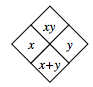### Home > AC > Chapter 1 > Lesson 1.1.3 > Problem1-21

1-21.

Copy these Diamond Problems and use the pattern you discovered earlier, shown at right, to complete each of them. Some of these may be challenging!1.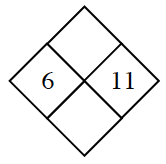For Diamond Problems where you have the right and left numbers, simply find their product to fill in the top space and their sum for the bottom space.

1.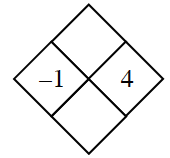Make sure not to forget about the negative sign! The product of a positive and negative number should be negative.

$−1·4=−4$

$−1+4=3$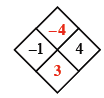1.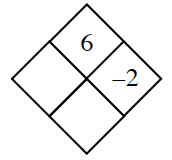Remember that a negative number must be multiplied by another negative number in order to have a positive product.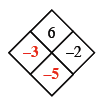1.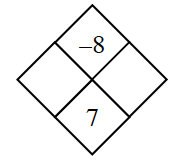Try listing the factors of the top number. Which pair of factors result in the bottom number when added?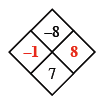1.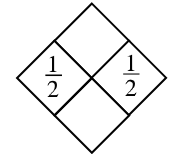When multiplying fractions, find the answer by writing the product of the numerators over the product of the denominators.

1.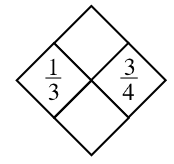What is the lowest common denominator?

$\frac{1}{3} \cdot \frac{3}{4}=\frac{1\cdot 3}{3\cdot 4}=\frac{3}{12}=\frac{1}{4}$

$\frac{1}{3}\cdot\frac{4}{4}+\frac{3}{4}\cdot\frac{3}{3}=\frac{4}{12}+\frac{9}{12}=\frac{13}{12}$

1.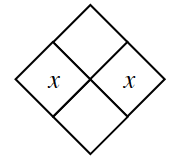Is there a better way to write the product than $x·x$?

1.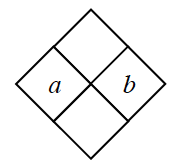See the example below.